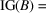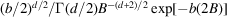International
Tables for
Crystallography
Volume F
Crystallography of biological macromolecules
Edited by E. Arnold, D. M. Himmel and M. G. Rossmann

International Tables for Crystallography (2012). Vol. F, ch. 18.4, p. 487   | 1 | 2 |

Figure 18.4.1.2

Z. Dauter,a G. N. Murshudovb and K. S. Wilsonb*

aNCI Frederick & Argonne National Laboratory, Building 202, Argonne, IL 60439, USA, and bYork Structural Biology Laboratory, Department of Chemistry, University of York, York YO10 5YW, UK
Correspondence e-mail:  keith@ysbl.york.ac.ukFigure 18.4.1.2 Histograms of B values for a protein structure, Micrococcus lysodecticus catalase (Murshudov et al., 1999), for two different crystals which diffracted to different limiting resolutions. For both crystals, the resolution cutoff reflects the real diffraction limit from the sample, and hence its level of order. At 0.89 Å, the mean B value is 8.3 Å2 and the width of the distribution is small. In contrast, at 1.96 Å, the mean B value is 25.5 Å2 and the spread is correspondingly large. Thus, for the 0.89 Å crystal, most atoms contribute to the high-resolution terms, whereas for the 1.96 Å crystal, only the atoms with lower B values do so. The thin line shows the theoretical inverse gamma distribution, where b and d are the parameters of the distribution, and Γ is the gamma function. For this figure, the valuesandwere chosen, which correspond to a mean B value of 20 Å2 andof 11 Å2. In the gamma distribution, the abscissa was multiplied by 8π2 to make it comparable with the measured B values. All three histograms were normalized to the same scale.